Habeebpasha Makandar

•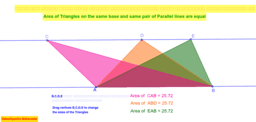Area of Triangles on same base between same pair of parallel lines

Activity

Habeebpasha Makandar

•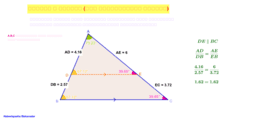Thales Theorem

Activity

Habeebpasha Makandar

•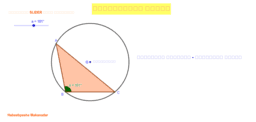Position of CircumCentre

Activity

Habeebpasha Makandar

•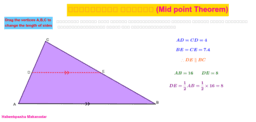Mid Point Theorem

Activity

Habeebpasha Makandar

•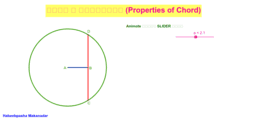Properties of Chord

Activity

Habeebpasha Makandar

•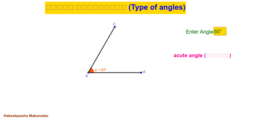Types of Angles

Activity

Habeebpasha Makandar

•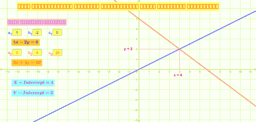Pair of Linear eq'ns in Two Variables

Activity

Habeebpasha Makandar

•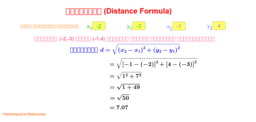Distance formula-Co ordinate Geometry

Activity

Habeebpasha Makandar

•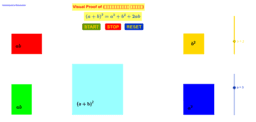(a+b)^2=a^2+b^2+2ab

Activity

Habeebpasha Makandar

•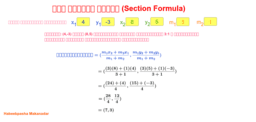Section formula-Co ordinate Geometry

Activity

Habeebpasha Makandar

•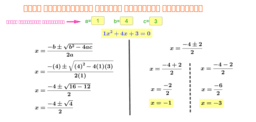Activity

Habeebpasha Makandar

•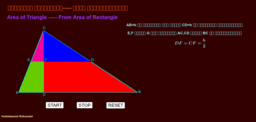Area of triangle from area of rectangle

Activity

Habeebpasha Makandar

•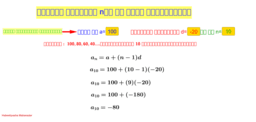nth term of an A.P

Activity

Habeebpasha Makandar

•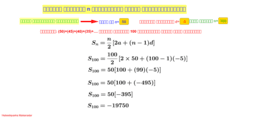Sum of 'n' terms of an A.P(Series)

Activity

Habeebpasha Makandar

•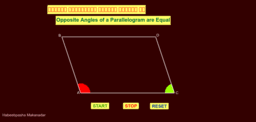Opposite Angles of a Parallelogram are Equal

Activity

Habeebpasha Makandar

•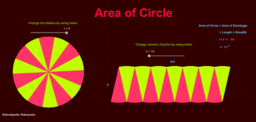Area of Circle

Activity

Habeebpasha Makandar

•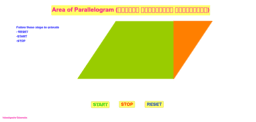Area of Parallelogram

Activity

Habeebpasha Makandar

•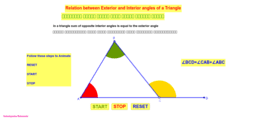Relation Between Exterior and Interior angles of a Triangle

Activity

Habeebpasha Makandar

•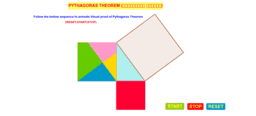Pythagoras Theorem

Activity

Habeebpasha Makandar

•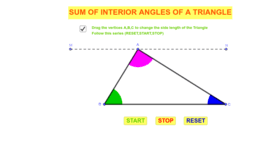Sum of Interior Angles of a Triangle

Activity

Habeebpasha Makandar

•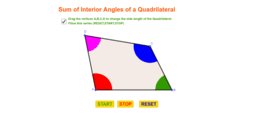Sum of Interior Angles of a Quadrilateral

Activity

Habeebpasha Makandar